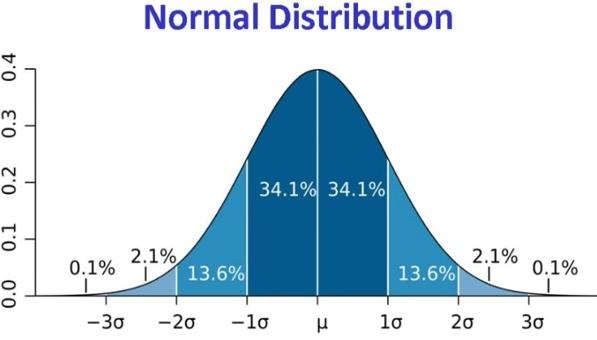# InfoCoBuild

## Shaping Modern Mathematics

By Professor Raymond Flood. The 19th Century saw the development of a mathematics profession with people earning their living from teaching, examining and researching and with the mathematical centre of gravity moving from France to Germany. A lot of the mathematics taught at university today was initiated at that time. Whereas in the 18th Century one would use the term 'mathematician', by the end of the 19th Century one had specialists in analysis, algebra, geometry, number theory, probability and statistics, and applied mathematics. This series of lectures looks at the shaping of mathematical areas and at the people who were involved.

Professor Raymond Flood is Emeritus Fellow and a member of the Continuing Education Department at Kellogg College, Oxford, and is Professor of Geometry at Gresham College. (from gresham.ac.uk)Ghosts of Departed Quantities: Calculus and its Limits
In 1734 Bishop Berkeley published a witty and effective attack on the foundations of the calculus as developed by Newton and Leibniz.

Polynomials and their Roots
We are familiar with the formula for solving a quadratic equation where the highest power of the unknown is a square.

From One to Many Geometries
For 100 years up to the end of the 19th century the study of geometry was completely changed with the development of non-Euclidean geometries and the use of techniques to think of geometries in higher dimensions.

The Queen of Mathematics
Carl Friedrich Gauss one of the greatest mathematicians, is said to have claimed: "Mathematics is the queen of the sciences and number theory is the queen of mathematics."

Are Averages Typical?
Not necessarily, for example the average person has fewer than two legs! This is because some people have fewer than two legs but nobody has more than two, so dividing the total number of legs by the total number of people to get the average gives a number less than two.

Modeling the World
An area of which 19th century British mathematics could be uniformly proud was applied mathematics where new techniques were used on a wide range of problems.

 Related Links Great Mathematicians, Great Mathematics This is a series of lectures to look at the personality and some of the work of a number of famous mathematicians and then discuss more recent developments and applications. Applying Modern Mathematics Professor Raymond Flood gives a series of lectures on the development of mathematics in the twentieth century. History of Mathematics This course provides an overview of the history of mathematics from the ancient Greek mathematics to the modern one. The Story of Maths This is a BBC documentary series written and presented by Professor Marcus du Sautoy, outlining aspects of the history of mathematics. Mathematics: Making the Invisible Visible Often described as the science of patterns, mathematics is arguably humanity's most penetrating mental framework for uncovering the hidden patterns that lie behind everything we see, feel, and experience.